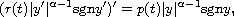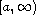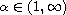Electron. J. Differential Equations, Vol. 2016 (2016), No. 267, pp. 1-27.

### Methods in half-linear asymptotic theory Pavel Rehak

Abstract:
We study the asymptotic behavior of eventually positive solutions of the second-order half-linear differential equationwhere r(t) and p(t) are positive continuous functions on,. The aim of this article is twofold. On the one hand, we show applications of a wide variety of tools, like the Karamata theory of regular variation, the de Haan theory, the Riccati technique, comparison theorems, the reciprocity principle, a certain transformation of dependent variable, and principal solutions. On the other hand, we solve open problems posed in the literature and generalize existing results. Most of our observations are new also in the linear case.

Submitted August 14, 2015. Published October 7, 2016.
Math Subject Classifications: 34C11, 34C41, 34E05, 26A12.
Key Words: Half-linear differential equation; nonoscillatory solution; regular variation; asymptotic formula.

Show me the PDF file (402 KB), TEX file for this article.Pavel Rehak Institute of Mathematics Czech Academy of Sciences Zizkova 22, CZ-61662 Brno, Czech Republic email: rehak@math.cas.cz

Return to the EJDE web page